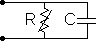Main >> Applications >> Sample problems

# ZnO lighting arrester

zinc oxide varistor simulation, electric FEA finite element analysis of the varistor, lightning stroke protection with varistor

This is an example of the ZnO arrester simulation, performed with QuickField software. The arrester consists of ZnO tablet placed inside ceramic tube. Electrodes are connected to the end tablets.

Problem Type
Axisymmetric problem of transient electric.

Geometry

Given
Relative permittivity of the air ε = 1.
Relative permittivity of the ceramic ε = 2.
Relative permittivity of ZnO element ε = 60.
Conductivity of ZnO element - nonlinear:

Nominal voltage U0 = 35 kV.
Maximal current Imax = 520 A.
Surge peak voltage Umax = 200 kV.

Calculate the varistor current.

Results
The arrester can be represented by the equivalent schema with resistor R and capacitance C connected in parallel.At nominal voltage the arrester acts as a capacitance (IC>IR). At overvoltage (surge) IR prevails and arrester acts like a resistor. QuickField can calculate both active (IR) and reactive (IC) currents.

 Voltage Active current 35 kV 0.67 mA 197 kV 433 A

Surge impulse passing through the arrester• Video: ZnO lighting arrester
• View on-line.
• View simulation report in PDF
• Download simulation files (files may be viewed using any QuickField Edition).
•